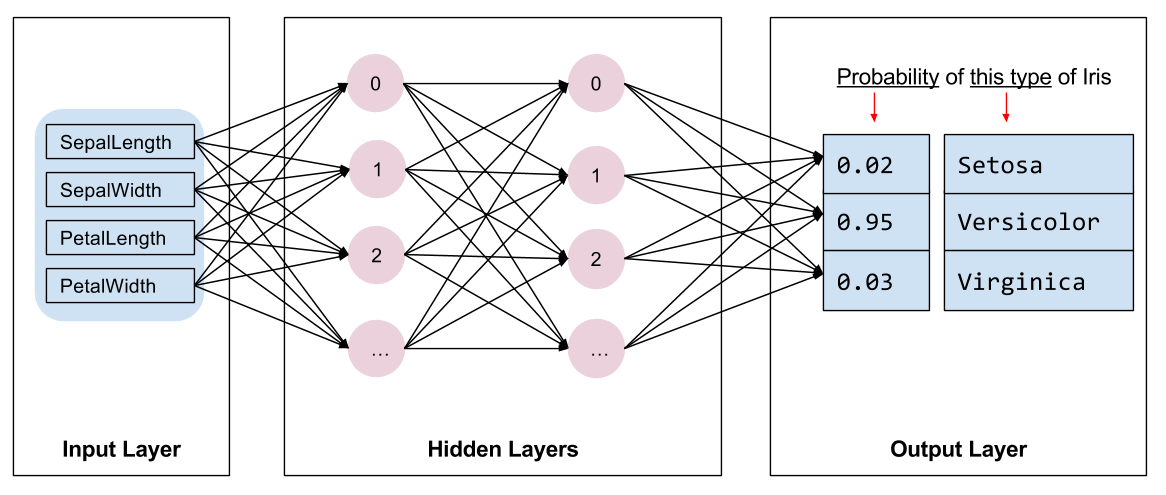1. 使用Keras演示TensorFlow2.0自定义训练实战 (tensorflow2.0官方教程翻译）

# 使用Keras演示TensorFlow2.0自定义训练实战 (tensorflow2.0官方教程翻译）

1. 构建模型
2. 使用样本数据训练该模型
3. 利用该模型对未知数据进行预测。

## 1. TensorFlow 编程

1. 导入和解析数据集。

2. 选择模型类型。

3. 训练模型。

4. 评估模型的效果。

5. 使用经过训练的模型进行预测。

## 2. 设置程序

### 2.1. 配置导入

from __future__ import absolute_import, division, print_function, unicode_literals

import os
import matplotlib.pyplot as plt

import tensorflow as tf

print("TensorFlow version: {}".format(tf.__version__))
print("Eager execution: {}".format(tf.executing_eagerly()))

      TensorFlow version: 2.0.0-alpha0 Eager execution: True


## 3. 鸢尾花分类问题

• 山鸢尾
• 维吉尼亚鸢尾
• 变色鸢尾图1. 山鸢尾Iris setosa, 变色鸢尾Iris versicolor，和 维吉尼亚鸢尾Iris virginica

## 4. 导入和解析训练数据集

### 4.1. 下载数据集

train_dataset_url = "https://storage.googleapis.com/download.tensorflow.org/data/iris_training.csv"

train_dataset_fp = tf.keras.utils.get_file(fname=os.path.basename(train_dataset_url),
origin=train_dataset_url)

print("Local copy of the dataset file: {}".format(train_dataset_fp))


### 4.2. 检查数据

!head -n5 {train_dataset_fp}

      120,4,setosa,versicolor,virginica
6.4,2.8,5.6,2.2,2
5.0,2.3,3.3,1.0,1
4.9,2.5,4.5,1.7,2
4.9,3.1,1.5,0.1,0


1. 第一行是标题，其中包含数据集信息：
• 共有 120 个样本。每个样本都有四个特征和一个标签名称，标签名称有三种可能。
1. 后面的行是数据记录，每个样本各占一行，其中：
• 前四个字段是特征：即样本的特点。在此数据集中，这些字段存储的是代表花卉测量值的浮点数。
• 最后一列是标签：即我们想要预测的值。对于此数据集，该值为 0、1 或 2 中的某个整数值（每个值分别对应一个花卉名称）。

# column order in CSV file
column_names = ['sepal_length', 'sepal_width', 'petal_length', 'petal_width', 'species']

feature_names = column_names[:-1]
label_name = column_names[-1]

print("Features: {}".format(feature_names))
print("Label: {}".format(label_name))

      Features: ['sepal_length', 'sepal_width', 'petal_length', 'petal_width'] Label: species


• 0: 山鸢尾
• 1: 变色鸢尾
• 2: 维吉尼亚鸢尾

class_names = ['Iris setosa', 'Iris versicolor', 'Iris virginica']


### 4.3. 创建一个 tf.data.Dataset

TensorFlow 的 Dataset API 可处理在向模型加载数据时遇到的许多常见情况。这是一种高阶 API，用于读取数据并将其转换为可供训练使用的格式。如需了解详情，请参阅数据集快速入门指南

batch_size = 32

train_dataset = tf.data.experimental.make_csv_dataset(
train_dataset_fp,
batch_size,
column_names=column_names,
label_name=label_name,
num_epochs=1)


make_csv_dataset 函数返回 (features, label) 对的 tf.data.Dataset，其中 features 是一个字典：{'feature_name': value}

features, labels = next(iter(train_dataset))

print(features)


plt.scatter(features['petal_length'],
features['sepal_length'],
c=labels,
cmap='viridis')

plt.xlabel("Petal length")
plt.ylabel("Sepal length")
plt.show()def pack_features_vector(features, labels):
"""Pack the features into a single array."""
features = tf.stack(list(features.values()), axis=1)
return features, labels


train_dataset = train_dataset.map(pack_features_vector)


Dataset 的 features 元素现在是形状为 (batch_size, num_features) 的数组。我们来看看前几个样本：

features, labels = next(iter(train_dataset))

print(features[:5])

    tf.Tensor(
[[4.9 2.4 3.3 1. ]
...
[6.6 3. 4.4 1.4]], shape=(5, 4), dtype=float32)


## 5. 选择模型类型

### 5.2. 选择模型图 2. 包含特征、隐藏层和预测的神经网络

### 5.3. 使用Keras创建模型

TensorFlow tf.keras API 是创建模型和层的首选方式。通过该 API，您可以轻松地构建模型并进行实验，而将所有部分连接在一起的复杂工作则由 Keras 处理。

tf.keras.Sequential 模型是层的线性堆叠。该模型的构造函数会采用一系列层实例；在本示例中，采用的是 2 个密集层（分别包含 10 个节点）以及 1 个输出层（包含 3 个代表标签预测的节点）。第一个层的 input_shape 参数对应该数据集中的特征数量，它是一项必需参数。

model = tf.keras.Sequential([
tf.keras.layers.Dense(10, activation=tf.nn.relu, input_shape=(4,)),  # input shape required
tf.keras.layers.Dense(10, activation=tf.nn.relu),
tf.keras.layers.Dense(3)
])


### 5.4. 查看模型

predictions = model(features)
predictions[:5]


tf.nn.softmax(predictions[:5])


print("Prediction: {}".format(tf.argmax(predictions, axis=1)))
print("    Labels: {}".format(labels))


## 6. 训练模型

### 6.1. 定义损失和梯度函数

loss_object = tf.keras.losses.SparseCategoricalCrossentropy(from_logits=True)

def loss(model, x, y):
y_ = model(x)

return loss_object(y_true=y, y_pred=y_)

l = loss(model, features, labels)
print("Loss test: {}".format(l))


def grad(model, inputs, targets):
loss_value = loss(model, inputs, targets)


### 6.2. 创建优化器图 3. 优化算法在三维空间中随时间推移而变化的可视化效果。(Source: 斯坦福大学 CS231n 课程, MIT License, Image credit: Alec Radford)

TensorFlow 拥有许多可用于训练的优化算法。此模型使用的是 tf.train.GradientDescentOptimizer，它可以实现随机梯度下降法 (SGD)。learning_rate 用于设置每次迭代（向下行走）的步长。这是一个超参数，您通常需要调整此参数以获得更好的结果。

optimizer = tf.keras.optimizers.Adam(learning_rate=0.01)


loss_value, grads = grad(model, features, labels)

print("Step: {}, Initial Loss: {}".format(optimizer.iterations.numpy(),
loss_value.numpy()))

print("Step: {},         Loss: {}".format(optimizer.iterations.numpy(),
loss(model, features, labels).numpy()))

      Step: 0, Initial Loss: 2.3108744621276855
Step: 1, Loss: 1.7618987560272217


### 6.3. 训练循环

1. 迭代每个周期。通过一次数据集即为一个周期。
2. 在一个周期中，遍历训练 Dataset 中的每个样本，并获取样本的特征 (x) 和标签 (y)。
3. 根据样本的特征进行预测，并比较预测结果和标签。衡量预测结果的不准确性，并使用所得的值计算模型的损失和梯度。
4. 使用 optimizer 更新模型的变量。
5. 跟踪一些统计信息以进行可视化。
6. 对每个周期重复执行以上步骤。

num_epochs 变量是遍历数据集集合的次数。与直觉恰恰相反的是，训练模型的时间越长，并不能保证模型就越好。num_epochs 是一个可以调整的超参数。选择正确的次数通常需要一定的经验和实验基础。

## Note: Rerunning this cell uses the same model variables

# keep results for plotting
train_loss_results = []
train_accuracy_results = []

num_epochs = 201

for epoch in range(num_epochs):
epoch_loss_avg = tf.keras.metrics.Mean()
epoch_accuracy = tf.keras.metrics.SparseCategoricalAccuracy()

# Training loop - using batches of 32
for x, y in train_dataset:
# Optimize the model

# Track progress
epoch_loss_avg(loss_value)  # add current batch loss
# compare predicted label to actual label
epoch_accuracy(y, model(x))

# end epoch
train_loss_results.append(epoch_loss_avg.result())
train_accuracy_results.append(epoch_accuracy.result())

if epoch % 50 == 0:
print("Epoch {:03d}: Loss: {:.3f}, Accuracy: {:.3%}".format(epoch,
epoch_loss_avg.result(),
epoch_accuracy.result()))

      Epoch 000: Loss: 1.568, Accuracy: 30.000%
...
Epoch 200: Loss: 0.049, Accuracy: 97.500%


### 6.4. 可视化损失函数随时间推移而变化的情况

fig, axes = plt.subplots(2, sharex=True, figsize=(12, 8))
fig.suptitle('Training Metrics')

axes.set_ylabel("Loss", fontsize=14)
axes.plot(train_loss_results)

axes.set_ylabel("Accuracy", fontsize=14)
axes.set_xlabel("Epoch", fontsize=14)
axes.plot(train_accuracy_results)
plt.show()## 7. 评估模型的效果

5.93.04.31.511
6.93.15.42.122
5.13.31.70.500
6.0 3.4 4.5 1.6 12
5.52.54.01.311

### 7.1. 设置测试数据集

test_url = "https://storage.googleapis.com/download.tensorflow.org/data/iris_test.csv"

test_fp = tf.keras.utils.get_file(fname=os.path.basename(test_url),
origin=test_url)

test_dataset = tf.data.experimental.make_csv_dataset(
test_fp,
batch_size,
column_names=column_names,
label_name='species',
num_epochs=1,
shuffle=False)

test_dataset = test_dataset.map(pack_features_vector)


### 7.2. 根据测试数据集评估模型

test_accuracy = tf.keras.metrics.Accuracy()

for (x, y) in test_dataset:
logits = model(x)
prediction = tf.argmax(logits, axis=1, output_type=tf.int32)
test_accuracy(prediction, y)

print("Test set accuracy: {:.3%}".format(test_accuracy.result()))

      Test set accuracy: 96.667%


tf.stack([y,prediction],axis=1)

      <tf.Tensor: id=164408, shape=(30, 2), dtype=int32, numpy=
array([[1, 1],
[2, 2],
[0, 0],..., dtype=int32)>


## 8. 使用经过训练的模型进行预测

• 0：山鸢尾
• 1：变色鸢尾
• 2：维吉尼亚鸢尾
predict_dataset = tf.convert_to_tensor([
[5.1, 3.3, 1.7, 0.5,],
[5.9, 3.0, 4.2, 1.5,],
[6.9, 3.1, 5.4, 2.1]
])

predictions = model(predict_dataset)

for i, logits in enumerate(predictions):
class_idx = tf.argmax(logits).numpy()
p = tf.nn.softmax(logits)[class_idx]
name = class_names[class_idx]
print("Example {} prediction: {} ({:4.1f}%)".format(i, name, 100*p))

      Example 0 prediction: Iris setosa (100.0%)
Example 1 prediction: Iris versicolor (100.0%)
Example 2 prediction: Iris virginica (99.5%)